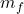## A polonium isotope with an atomic mass of 211.988868 u undergoes alpha decay, resulting in a daughter isotope with an atomic mass of 207.976

Question

A polonium isotope with an atomic mass of 211.988868 u undergoes alpha decay, resulting in a daughter isotope with an atomic mass of 207.976652 u. Ignoring any recoil of the daughter, find the kinetic energy of the emitted alpha particle in megaelectronvolts (MeV).

in progress 0
1 month 2021-08-12T13:00:14+00:00 1 Answers 0 views 0

K = 9.53 MeV

Explanation:

The kinetic energy that the alpha particle has emitted, is the energy in excess after removing the resting energy of the atoms and the helium nucleus that forms the alpha particle

Since energy and masses are related and cannot be

m₀ c² =c² + m_He c²+ K

K = c² (m₀ – m_{f} – m_He)

the mass of the Helium atom is 4 u

K = (3 10⁸)² (211,988868 -207.976652 – 4,002) 1,661 10⁻²⁷

K = 14,949 10⁻¹¹ (0.0102)

K = 1,527 10⁻¹² J

let’s reduce 1 J = 6,242 10¹² MeV

K = 9.53 MeV